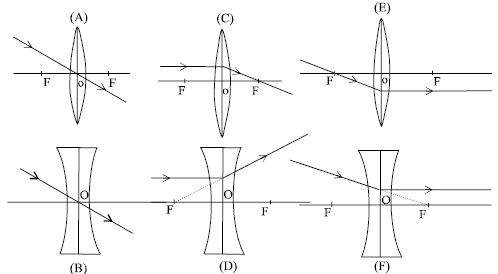Physics

# Rules of Drawing Ray Diagram in LensRules of Drawing Ray Diagram in Lens

• Rays of the light incident in a direction through the optical center of a lens are refracted along the same straight line without any deviation (Fig: A and B)
• Rays incident in a direction parallel to the principal axis after refraction passes through the principal focus (in the case of a convex lens) [fig (C)] or appear to diverge from the principal focus (in the case of a concave lens) [fig (D)].
• A ray of light incidents through the principal focus (in the case of a convex lens) [fig (E)] or directed towards the principal focus (in the case of a concave lens) [fig (F)] after refraction through the lens becomes parallel to the principal axis. Using a straight edge, accurately draw one ray so that it passes exactly through the focal point on the way to the lens. (As on figure).Rules for Drawing Ray Diagrams for Concave Lenses

Rule 1: Incident light rays that are parallel to the principal axis will appear to come from the opposite focal point after refracting through the lens.

Rule 2: Incident light rays that are directed at the optical center will pass directly through – in other words, they are not refracted.

Rule 3: Incident light rays that are directed at the opposite focal point will become parallel to the principal axis after refracting through the lens. (This is the reverse concept of rule 1.)

The principal focus of a convex lens (sometimes called the focal point) is the point on the principal axis, through which rays of light, traveling near to and parallel to the principal axis, pass after refraction by the lens.

Rules for Drawing Ray Diagrams for Convex Lenses

Rule 1: Incident light rays that are parallel to the principal axis will pass through the focal point after refracting through the lens.

Rule 2: Incident light rays that are directed at the optical center will pass directly through – in other words, they are not refracted.

Rule 3: Incident light rays that pass through the focal point before hitting the lens will become parallel to the principal axis after refracting through the lens. (This is the reverse concept of rule 1.)

The focal length of a lens is the distance between the pole of the lens and the principal focus. If the lens has a real focus then the focal length is positive. If it has a virtual focus it is negative.